Question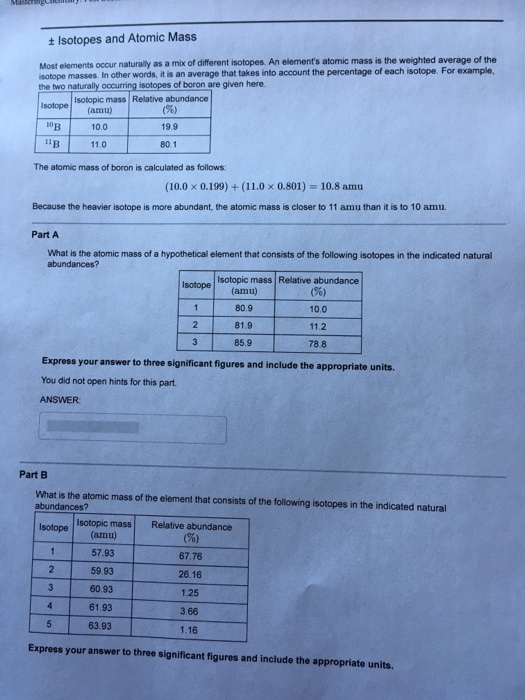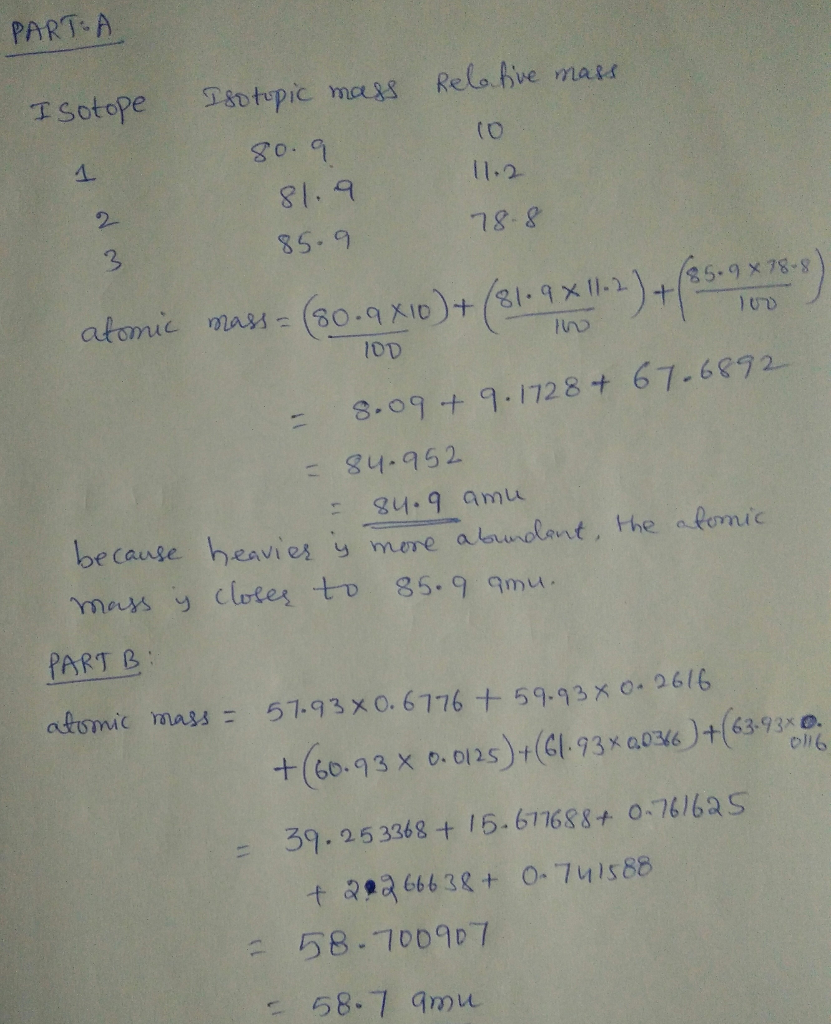#### Earn Coins

Coins can be redeemed for fabulous gifts.

Similar Homework Help Questions
• ### Part A What is the relative atomic mass of a hypothetical element that consists of the...

Part A What is the relative atomic mass of a hypothetical element that consists of the following isotopes in the indicated natural abundances? Isotope Isotopic mass (amu) Relative abundance (%) 1 81.9 12.2 2 84.9 15.0 3 88.9 72.8 Express your answer to three significant figures and include the appropriate units. Part B What is the relative atomic mass of the element that consists of the following isotopes in the indicated natural abundances? Isotope Isotopic mass (amu) Relative abundance (%)...

• ### Part A What is the relative atomic mass of a hypothetical element that consists of the...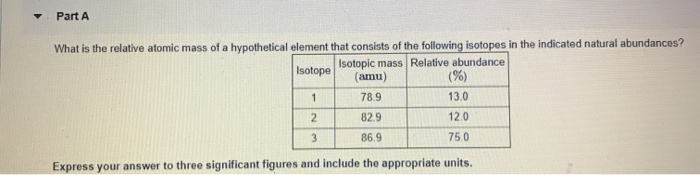Part A What is the relative atomic mass of a hypothetical element that consists of the following isotopes in the indicated natural abundances? Isotope Isotopic mass Relative abundance (amu) (%) 78.9 82.9 13.0 120 869 750 Express your answer to three significant figures and include the appropriate units.

• ### An element has two naturally-occurring isotopes. The mass numbers of these isotopes are 111 amu and...

An element has two naturally-occurring isotopes. The mass numbers of these isotopes are 111 amu and 113 amu, with natural abundances of 75% and 25%, respectively. Calculate its average atomic mass.

• ### Review Constants Periodic Table Part A Atomic mass is a weighted average of the masses of...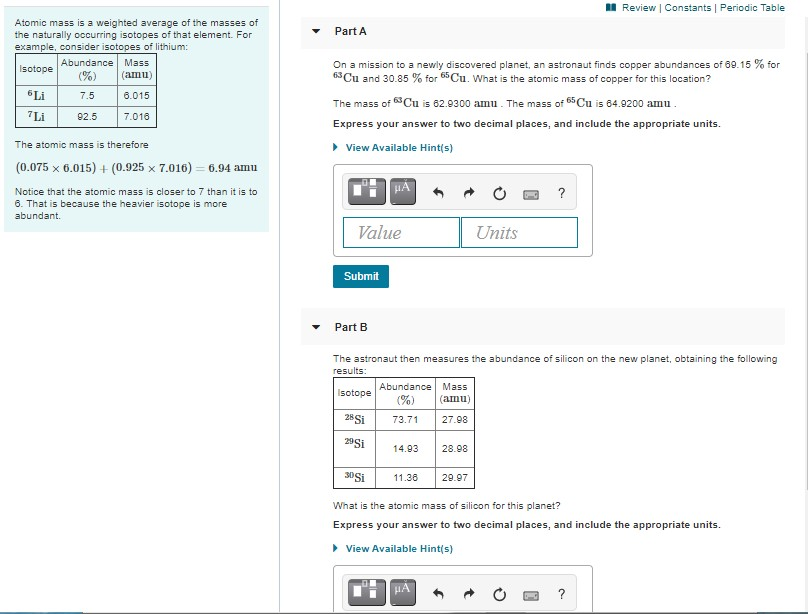Review Constants Periodic Table Part A Atomic mass is a weighted average of the masses of the naturally occurring isotopes of that element. For example, consider isotopes of lithium: Abundance Mass Isotope (%) (amu) Li 7.5 6.015 On a mission to a newly discovered planet, an astronaut finds copper abundances of 69.15 % for Cu and 30.85 % for 65Cu. What is the atomic mass of copper for this location? The mass of Cu is 62.9300 amu . The mass...

• ### An element has two naturally-occurring isotopes. The mass numbers of these isotopes are 125 amu and...

An element has two naturally-occurring isotopes. The mass numbers of these isotopes are 125 amu and 127 amu, with natural abundances of 80% and 20%, respectively. Calculate its average atomic mass. Report your answer to 1 decimal place. -------- amu

• ### 4. An element has two naturally occurring isotopes. The mass numbers of these isotopes are 111...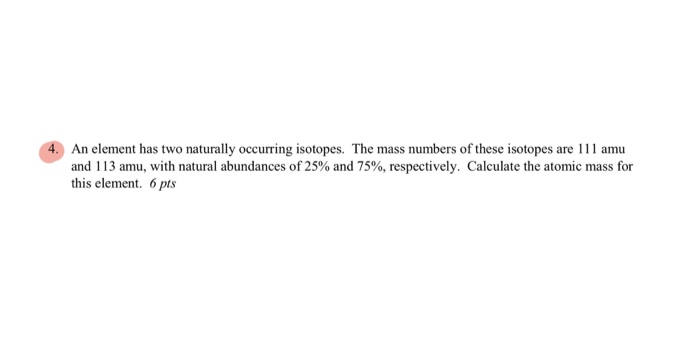4. An element has two naturally occurring isotopes. The mass numbers of these isotopes are 111 amu and 113 amu, with natural abundances of 25% and 75%, respectively. Calculate the atomic mass for this element. 6 pts

• ### UITGUIC Potassium has three naturally occurring isotopes. They are "K (93.26%, ? amu), "K (0.0117%, 39.964...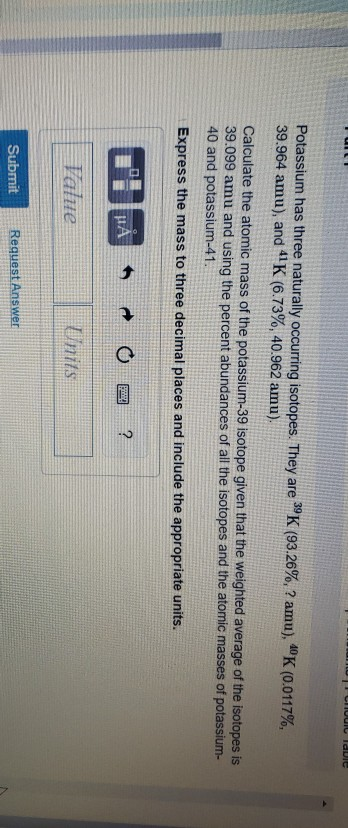UITGUIC Potassium has three naturally occurring isotopes. They are "K (93.26%, ? amu), "K (0.0117%, 39.964 amu), and 4K (6.73%, 40.962 amu) Calculate the atomic mass of the potassium-39 isotope given that the weighted average of the isotopes is 39.099 amu and using the percent abundances of all the isotopes and the atomic masses of potassium- 40 and potassium-41. Express the mass to three decimal places and include the appropriate units. Submit Request Answer

• ### The element carbon has two naturally occurring isotopes. The isotopic masses and abundances of these isotopes...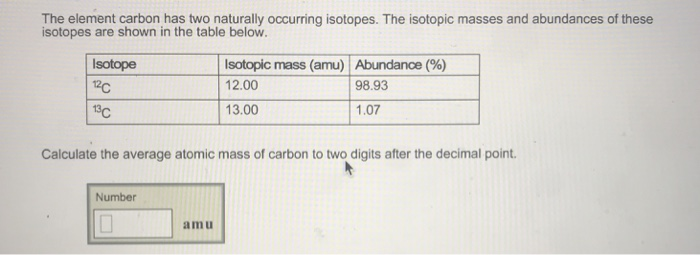The element carbon has two naturally occurring isotopes. The isotopic masses and abundances of these isotopes are shown in the table below. Isotope 12c isotopic mass (amu) Abundance (%) 12.00 13.00 98.93 1.07 Calculate the average atomic mass of carbon to two digits after the decimal point. Number = _______ amu

• ### Enter your answer in the provided box. An element has two naturally occurring isotopes. The mass...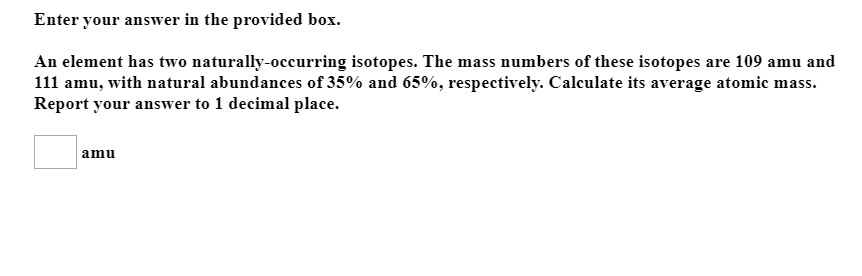Enter your answer in the provided box. An element has two naturally occurring isotopes. The mass numbers of these isotopes are 109 amu and 111 amu, with natural abundances of 35% and 65%, respectively. Calculate its average atomic mass. Report your answer to 1 decimal place. amu

• ### 5) Calculate the atomic mass of an imaginary element X that has 3 naturally occurring isotopes...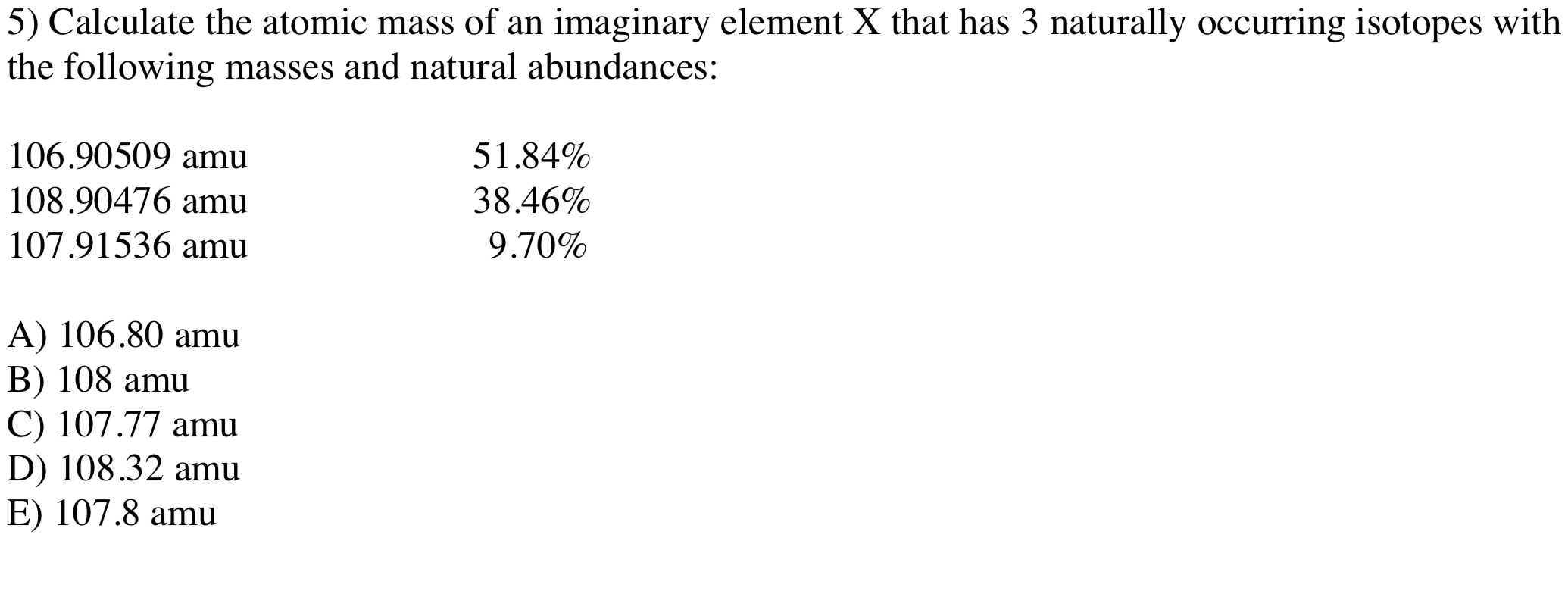5) Calculate the atomic mass of an imaginary element X that has 3 naturally occurring isotopes with the following masses and natural abundances: 106.90509 amu 108.90476 amu 107.91536 amu 51.84% 38.46% 9.70% A) 106.80 amu B) 108 amu C) 107.77 amu D) 108.32 amu E) 107.8 amu